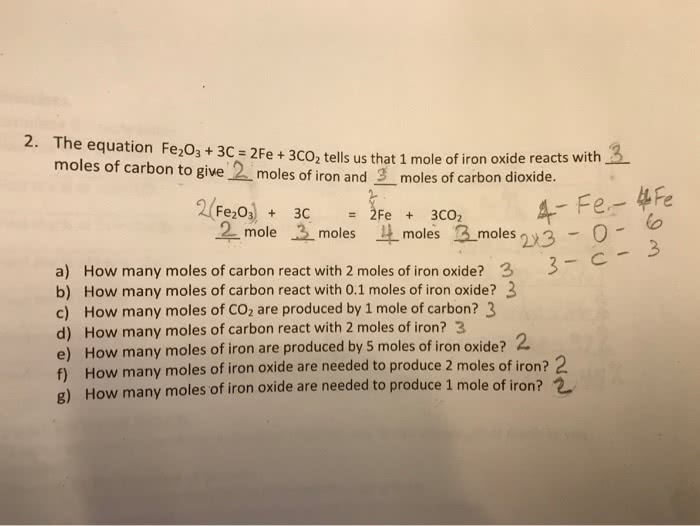1
0
watching
153
views18 Nov 2019

#xide reacts with moles of carbon to give 2 moles of iron and 3 moles of carbon dioxide 2 Fe o)+ 2 mole 4- Fe 0 é¼moles 3-moles 213 moles 3 a) How many moles of carbon react with 2 moles of iron oxide?3 b) How many moles of carbon react with 0.1 moles of iron oxide? 3 c) How many moles of CO2 are produced by 1 mole of carbon? 3 d) How many moles of carbon react with 2 moles of iron? 3 e) How many moles of iron are produced by 5 moles of iron oxide? 2 f) How many moles of iron oxide are needed to produce 2 moles of iron? 2 g) How many moles of iron oxide are needed to produce 1 mole of ir on?